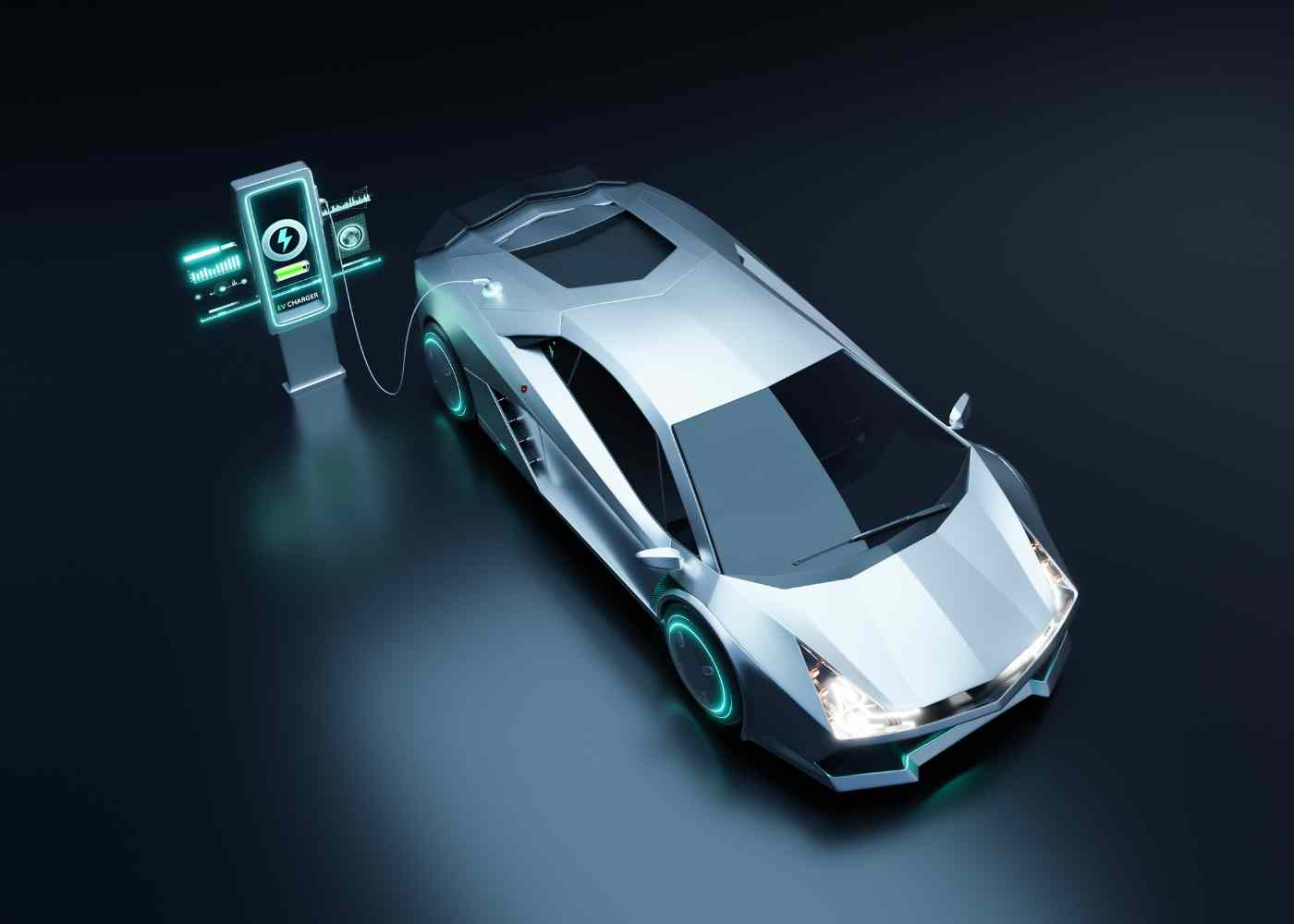Hot Articles:

# How Much Does It Cost to Charge An Electric Car?

##### Reverbtime Magazine -
• 0
• 645
Scroll Down For More

The cost of charging an electric car can vary significantly depending on several factors, including your location, the type of charger you use, your electricity rates, and the specific electric vehicle you own. Here are some key factors to consider when determining the cost of charging an electric car:

Beneath the sleek exteriors and futuristic promise, how much does it truly cost to charge an electric car? Is it a pocket-friendly alternative to traditional gasoline vehicles? We're here to untangle the numbers and find out.### How do you calculate the cost of charging an electric car?

Calculating the cost of EV charging is a relatively simple process using the formula provided:

Charging cost = (VR / RPK) x CPK

Let's break down the formula step by step and illustrate it with an example:

Vehicle range (VR): This refers to how far your electric car can go on a full charge. For instance, if your electric car has a range of 200 miles on a full charge, VR would be 200 miles.

Range per kWh (RPK): This factor estimates how many miles you can drive per kilowatt-hour of electricity. On average, this is typically around 3 to 4 miles per kWh. For this example, let's use 3 miles per kWh.

Cost per kWh (CPK): This is the cost of electricity per kilowatt-hour. Depending on where you charge your EV, CPK can vary. For this illustration, let's consider a Level 2 charger that costs \$0.23 per kWh.Now, let's calculate the monthly charging cost:

Charging cost = (VR / RPK) x CPK

Charging cost = (200 miles / 3 miles per kWh) x \$0.23 per kWh

Charging cost = (67 kWh) x \$0.23 per kWh

Charging cost = \$15.41

So, for a monthly driving distance of 1,200 miles, if you use a Level 2 charger that costs \$0.23 per kWh, your monthly charging cost would be around \$92.

It's important to note that this is a simplified calculation and actual costs may vary due to factors like fluctuations in electricity rates, driving conditions, and charging efficiency. However, this formula provides a good estimate of your monthly charging expenses based on your vehicle's range, the efficiency of your EV, and the cost of electricity in your area.

Related Posts